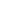# Ancient Mathematics: India

Ancient Indian mathematicians a proposed positional decimal system that was developed into contemporary decimal numerals. They calculated precise geometrical constructions for sacrificial altars in forms of square, circle, semicircle, isosceles triangle, and isosceles trapezoid, as described in Sulba Sutras (7th-3rd c BCE). Indian mathematicians introduced combinatorics around 4th c BCE. Starting from the 5th c CE, they introduced trigonometry, and since the 6th c CE considered negative solutions for square equations. In the early 16th c, they introduced series for functions sin x, cos x, and arctg x, and performed accurate trigonometric calculations two centuries before European mathematicians.

• Face-to-Face

#### SUGGESTED READING

• T. S. Bhanu Murthy. A Modern Introduction to Ancient Indian Mathematics. New Age International, 1993

#### COURSE OUTLINE

• Origin and development of Indian decimal numeral systems
• Sulba Sutras
• Drawing of a square, half area square, rectangle
• Areas of a circle, semicircle, isosceles triangle, and isosceles trapezoid
• Approximations for Pi
• Jain mathematics: calculations of circumference and segments
• The beginnings of trigonometry
• Introducing combinatorics
• Negative solutions for square equations
• Madhava and the Kerala School of Mathematics (14 c CE)
• Series for trigonometric functions

#### PLANNED LEARNING OUTCOMES

By the end of this course, students should be able to:

1. Know origin of contemporary decimal numeral system from Indian Brahmi numbers.
2. Know main names of Ancient Indian mathematicians.
3. Know basic mathematical problems described in Sulba Sutras.
4. Know basics of Jain mathematics.
5. Know origins of the trigonometric terms.
6. Acquaintance with the achievements of the Kerala School of Mathematics.
\$65 Limited

<p>Ancient Indian mathematicians a proposed positional decimal system that was developed into contemporary decimal numerals. They calculated precise geometrical constructions for sacrificial altars in

...
23 Jul
Mo Tu We Th Fr Sa SuInterested in this course but can't attend? Please join the waiting list by clicking .